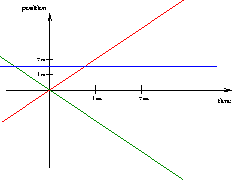Next: Free fall motion Up: Motion Previous: Acceleration

# Graphing Motion

It is often useful to represent the motion of objects pictorially on a position vs time graph. Such a graph takes the form of a line. Each point on the line specifies the position (relative to the origin) of an object at a given instant in time. The simplest motion'' is that of a stationary object, whose velocity and acceleration are zero. Since the position of such an object doesn't change its position vs time graph is a straight, horizontal line, as shown in blue in the Figure below.The next simplest motion, is that of an object going at constant velocity (hence zero acceleration). Its position vs time graph is again a straight line, but with non-zero slope. In fact the slope of the line (the rise over the run) equals the speed of the object. The red line in Fig.2.2 shows the position time graph of an object moving at 2 m/s along the positive x-axis. Since it is a straight line, the slope can be calculated between any two points on the graph. If the object were moving with constant velocity along the negative x-axis, the line would be sloped in the opposite direction: the value of the x-coordinate would decrease as time increased. This is illustrated by the green line.Next: Free fall motion Up: Motion Previous: Acceleration
modtech@theory.uwinnipeg.ca
1999-09-29